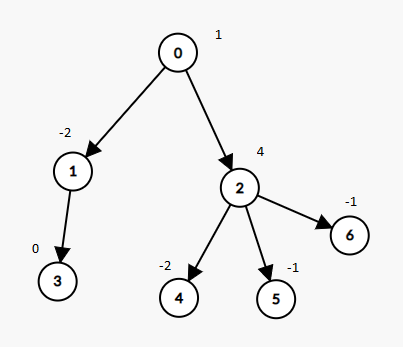##### Welcome to Subscribe On Youtube

Formatted question description: https://leetcode.ca/all/1273.html

# 1273. Delete Tree Nodes

Medium

## Description

A tree rooted at node 0 is given as follows:

• The number of nodes is nodes;
• The value of the i-th node is value[i];
• The parent of the i-th node is parent[i].

Remove every subtree whose sum of values of nodes is zero.

After doing so, return the number of nodes remaining in the tree.

Example 1:Input: nodes = 7, parent = [-1,0,0,1,2,2,2], value = [1,-2,4,0,-2,-1,-1]

Output: 2

Constraints:

• 1 <= nodes <= 10^4
• -10^5 <= value[i] <= 10^5
• parent.length == nodes
• parent == -1 which indicates that 0 is the root.

## Solution

According to parent, obtain each node’s children. Do breadth first search starting from the root and store all the nodes into a list in the order that they are visited. Then loop over the list in reversing order so that the leaf nodes are met first and the root is met last. During the loop, add each node’s value to its parent’s value. If a node’s value is 0 (including the case when its value becomes 0 after adding its children’s values), then the subtree with the node as the root has sum of values 0, so add the node to another list where nodes will be deleted. Use a set to store the nodes that are deleted. Do breadth first search starting from the nodes to be deleted, and add all the nodes and the nodes in the subtrees (the nodes are the roots of the subtrees) to the set. Finally, subtract the set’s size from nodes to obtain the number of nodes remaining in the tree.

• class Solution {
public int deleteTreeNodes(int nodes, int[] parent, int[] value) {
Map<Integer, List<Integer>> parentChildrenMap = new HashMap<Integer, List<Integer>>();
for (int i = 0; i < nodes; i++) {
int parentIndex = parent[i];
if (parentIndex >= 0) {
List<Integer> children = parentChildrenMap.getOrDefault(parentIndex, new ArrayList<Integer>());
parentChildrenMap.put(parentIndex, children);
}
}
List<Integer> nodesList = new ArrayList<Integer>();
queue.offer(0);
while (!queue.isEmpty()) {
int node = queue.poll();
List<Integer> children = parentChildrenMap.getOrDefault(node, new ArrayList<Integer>());
for (int child : children)
queue.offer(child);
}
List<Integer> deleteNodesList = new ArrayList<Integer>();
for (int i = nodes - 1; i >= 0; i--) {
int node = nodesList.get(i);
int parentIndex = parent[node];
if (value[node] == 0)
else if (parentIndex >= 0)
value[parentIndex] += value[node];
}
Set<Integer> deleteNodesSet = new HashSet<Integer>();
for (int i = deleteNodesList.size() - 1; i >= 0; i--)
deleteQueue.offer(deleteNodesList.get(i));
while (!deleteQueue.isEmpty()) {
int node = deleteQueue.poll();
List<Integer> children = parentChildrenMap.getOrDefault(node, new ArrayList<Integer>());
for (int child : children)
deleteQueue.offer(child);
}
}
return nodes - deleteNodesSet.size();
}
}

• // OJ: https://leetcode.com/problems/delete-tree-nodes/
// Time: O(N)
// Space: O(N)
class Solution {
vector<vector<int>> G;
pair<int, int> dfs(int u, vector<int> &value) {
int sum = value[u], cnt = 1;
for (int v : G[u]) {
auto [c, s] = dfs(v, value);
sum += s;
cnt += c;
}
if (sum == 0) cnt = 0;
return { cnt, sum };
}
public:
int deleteTreeNodes(int nodes, vector<int>& parent, vector<int>& value) {
G.assign(nodes, {});
for (int i = 1; i < nodes; ++i) {
G[parent[i]].push_back(i);
}
return dfs(0, value).first;
}
};

• class Solution:
def deleteTreeNodes(self, nodes: int, parent: List[int], value: List[int]) -> int:
def dfs(i):
s, m = value[i], 1
for j in g[i]:
t, n = dfs(j)
s += t
m += n
if s == 0:
m = 0
return (s, m)

g = defaultdict(list)
for i in range(1, nodes):
g[parent[i]].append(i)
return dfs(0)


• func deleteTreeNodes(nodes int, parent []int, value []int) int {
g := make([][]int, nodes)
for i := 1; i < nodes; i++ {
g[parent[i]] = append(g[parent[i]], i)
}
type pair struct{ s, n int }
var dfs func(int) pair
dfs = func(i int) pair {
s, m := value[i], 1
for _, j := range g[i] {
t := dfs(j)
s += t.s
m += t.n
}
if s == 0 {
m = 0
}
return pair{s, m}
}
return dfs(0).n
}